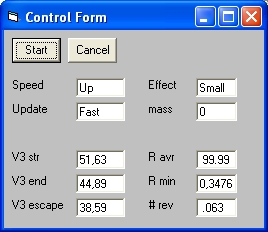## Visual Basic 5.0 Program: "VB kuiper"

The program VB Kuiper uses the standard Newton's Law to calculate the trajectories of 3 objects based on observations written in Visual Basic 5.0.
Newton's Law assumes that the sum of all the forces of all objects is zero.
Newton's Law law describes that the force between two objects is "equal" to the mass m1 times the mass m2 of each object divided by the distance. Because this force acts twice but in opposite directions as such the sum is zero. Because this is true for all objects and all forces the sum of all forces is zero.

For a more technical explanation about what kuiper belt binaries are, read this:> QBasic Program 20: Kuiper belt binaries

### VB Program: "VB Kuiper" Operation

The program uses two displays or forms: "Slingshot Control Form" and "Slingshot Simulation Display"### Slingshot Control Display

The VB program is controlled by three Commands:
• "Select" This Command is used to select the parameter values.
• "Start" This Command is used to start the simulation.
• "Cancel" This Command is used to End the execution of the Visual Basic program.

### VB Program: "VB Kuiper" executable

In order to get the Visual Basic 5.0 executable program use the following zip file:VB Kuiper.zip

The Visual Basic Executable program requires MSVDVM50.DLL: Msvdvm50.dll.zip

There is also a QBASIC program "kuiper.bas" available to demonstrate the kuiper-belt binaries. For a description select: kuiperbb.htm.
In order to get a copy of the program "kuiper.bas" select: kuiper.zip. This zip file also contains the executable: "kuiper.exe"